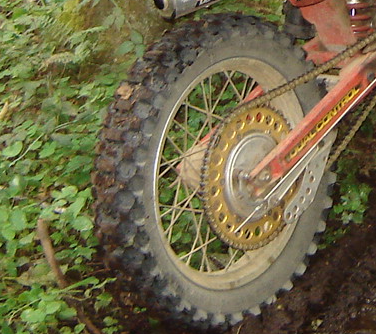Sunday, March 26, 2023 | TSTM

## Tire MathematicsThe speed at which your tire rotates and the size of your tires determines how fast you are able to move. You can compute the amount of ground your tire would cover in a single rotation by computing the circumference of a circle. PI * Diameter = Circumference so with a tire of 18 inches you would get 3.14 * 18 inches = 56.52 inches or 4.71 feet. This is how far your bike will move after the tire has rotated a full turn.

This can be further used to determine how many times the tire needs to rotate to cover an entire mile. There are 5,280 feet in a mile and your tire coverse 4.71 feet for every rotation so 5,280/4.71 feet = 1,121 rotations of the rear tire covers one mile. Please note that these are just estimates.

This means that to go one mile an hour the tire would need to rotate 1,121 times every hour or if you went 60 miles an hour it would need to rotate 1,121 times every minute. The math can now be related back to sprocket math that was discussed in the related article. This can be used to calculate how many times your drive shaft would need to rotate in relation to your tire.

So for example if you have a 15 tooth sprocket on your drive shaft and you have a 51 tooth sprocket on your rear tire that means you have a gear ratio of 51/15 = 1:3.4. This means that it takes 3.4 revolutions of the drive shaft to rotate the tire 1 full rotation, which is 4.71 feet. The math of 3.4 * 1,121 = 3,811 which is how many revolutions of your drive shaft it takes to go 1 mile.

This can be traced back through your transmission and eventually to your crank shaft to determine how many RPMs at any particular gear it takes to go 1 mile.

How much difference can one inch make? 3.14 * 19 inches = 59.66 or 4.97 feet. We can then calculate that it takes (5,280/4.97 = 1062) 1062 tire rotations to move a mile. The same sprockets can then calculate that (3.4 * 1062 = 3610) it would take 3610 rotations of the drive shaft to move a mile instead of 3,811. We can calculate then the speed gained by taking the original rotations of the drive shaft which are 3,811 rotations and dividing it by the number of rotations of the drive shaft to each rotation of the rear tire. 3,811/3.4 = 1120 rotations.

You will notice that this is the same number as before because the math didn't change and the number of rotations that the drive shaft moves along with the tire also didn't change. The only thing that changed was the distance the tire moves for each rotation. 4.97 feet * 1120 rotations = 5,566 feet. The math now indicates that the bike is now (5,566 feet / 5,280 feet = 1.054 miles) 0.054 miles faster at the same drive shaft rotation with the larger tire.

Please note that an 18" tire really isn't 18" that's the size of the rim. The tire itself may be closer to 26" so the above was simply an example you would need to do your own measurements if you wish to estimate how a change in tire size or sprockets would affect your speed at any given gear/RPM.